25 Ways to Apply Functions
f@x is the same as f[x]:
 In:=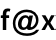Out=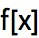In:=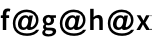Out=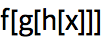In:=Out=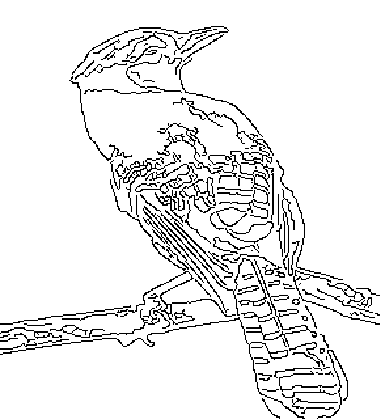Apply f as an afterthought to x:
 In:=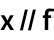Out=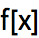You can have a sequence of afterthoughts:
 In:=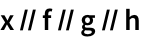Out=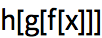In:=Out=A particularly common use of // is to apply N (for numerical evaluation) as an afterthought.
Apply numerical evaluation as an afterthought:
 In:=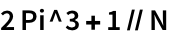Out=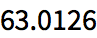Apply f to each element in a list:
 In:=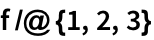Out=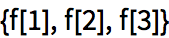f usually would just get applied to the whole list:
 In:=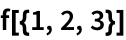Out=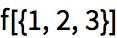Framed is a function that displays a frame around something.
Display x framed:
 In:=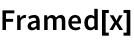Out=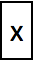Applying Framed to a list just puts a frame around the whole list.
Apply Framed to a whole list:
 In:=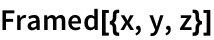Out=@ does exactly the same thing:
 In:=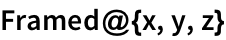Out=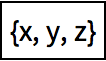Now use /@ to apply Framed to each element in the list:
 In:=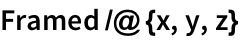Out=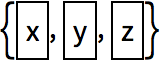The same thing works with any other function. For example, apply the function Hue separately to each number in a list.
/@ applies Hue separately to each number in the list:
 In:=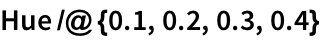Out=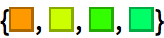Heres what the /@ is doing:
 In:=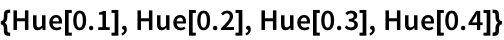Out=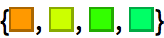Its the same story with Range, though now the output is a list of lists.
/@ applies Range separately to each number, producing a list of lists:
 In:=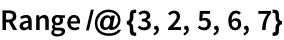Out=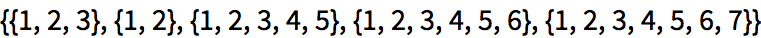Heres the equivalent, all written out:
 In:=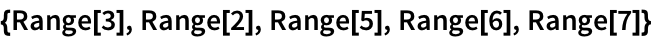Out=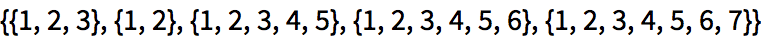Given a list of lists, /@ is what one needs to do an operation separately to each sublist.
Apply PieChart separately to each list in a list of lists:
 In:=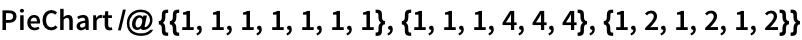Out=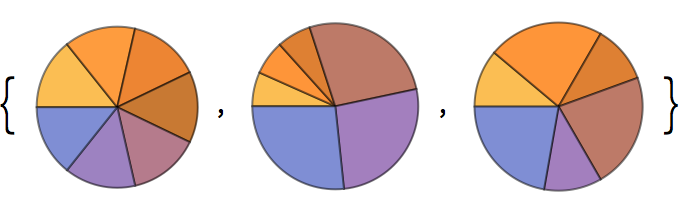Apply Length to each element, getting the length of each sublist:
 In:=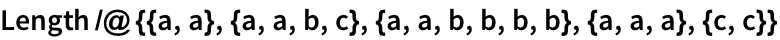Out=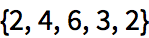Applying Length to the whole list just gives the total number of sublists:
 In:=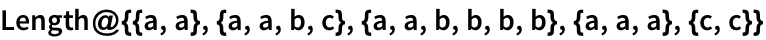Out=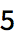Apply Reverse to each element, getting three different reversed lists:
 In:=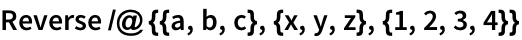Out=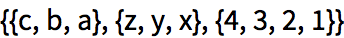Apply Reverse to the whole list, reversing its elements:
 In:=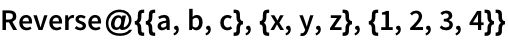Out=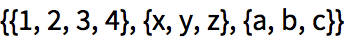As always, the form with brackets is exactly equivalent:
 In:=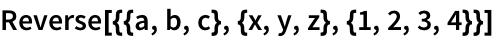Out=N is listable, so you dont have to use /@ to get it applied to each element in a list:
 In:=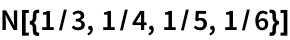Out=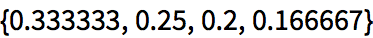In:=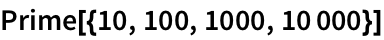Out=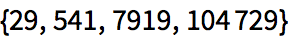A function like Graphics definitely isnt listable.
This makes a single graphic with three objects in it:
 In:=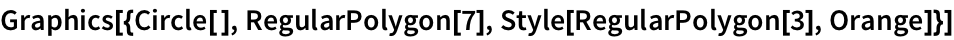Out=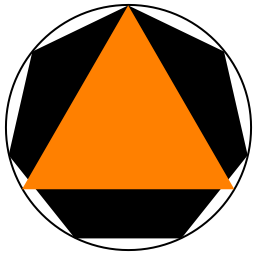This gives three separate graphics, with Graphics applied to each object:
 In:=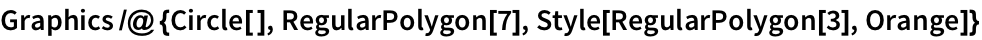Out=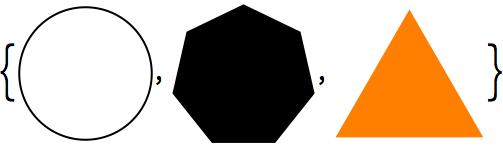When you enter f/@{1,2,3}, the Wolfram Language interprets it as Map[f,{1,2,3}]. f/@x is usually read as map f over x.
The internal interpretation of f/@{1, 2, 3}:
 In:=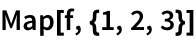Out=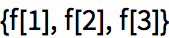f@x equivalent to f[x] x//f equivalent to f[x] f/@{a,b,c} apply f separately to each element of the list Map[f,{a,b,c}] alternative form of /@ Framed[expr] put a frame around something
25.1Use /@ and Range to reproduce the result of Table[f[n], {n, 5}]»
Expected output:
 Out[]=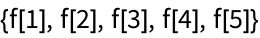Answer & check your solution
25.2Use /@ twice to generate Table[f[g[n]], {n, 10}]»
Expected output:
 Out[]=Answer & check your solution
25.3Use // to create a[b[c[d[x]]]]»
Expected output:
 Out[]=Answer & check your solution
Expected output:
 Out[]=Answer & check your solution
Expected output:
 Out[]=Answer & check your solution
25.6Use /@ to draw separate maps of each country in the G5. »
Expected output:
 Out[]=Answer & check your solution
25.7Binarize each flag in Europe, and make an image collage of the result. »
Expected output:
 Out[]=Answer & check your solution
25.8Find a list of the dominant colors in images of the planets, putting the results for each planet in a column. »
Expected output:
 Out[]=Answer & check your solution
25.9Find the total of the letter numbers given by LetterNumber for the letters in the word wolfram»
Expected output:
 Out[]=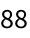Answer & check your solution
Why not always use f@x instead of f[x]?
f@x is a fine equivalent to f[x], but the equivalent of f[1+1] is f@(1+1), and in that case, f[1+1] is shorter and easier to understand.
Why is /@ called Map?
It comes from math. Given a set {1, 2, 3}, f/@{1, 2, 3} can be thought of as mapping of this set to another one.
How does one say "//" and "/@"?
Its determined by the precedence or binding of different operators. @ binds tighter than +, so f@1+1 means f+1 not f@(1+1) or f[1+1]. // binds looser than +, so 1/2+1/3//N means (1/2+1/3)//N. In a notebook you can find how things are grouped by repeatedly clicking on your input, and seeing how the selection expands.